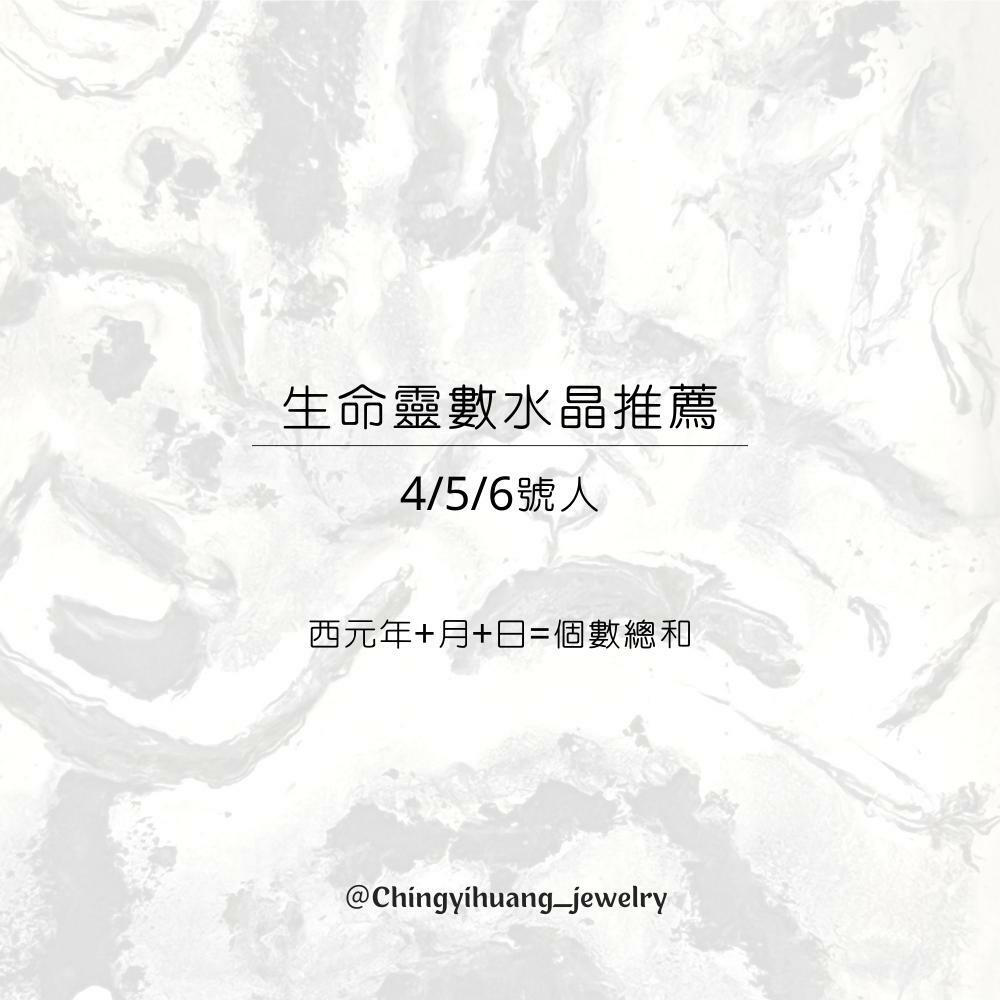A. 1988/01/01= 1+9+8+8+0+1+0+1=28
2+8=10
1+0=1號人

B. 1993/05/12= 1+9+9+3+0+5+1+2=30
3+0=3
3+0=3號人

C. 1995/03/15= 1+9+9+5+0+3+1+5=33
3+3=6號人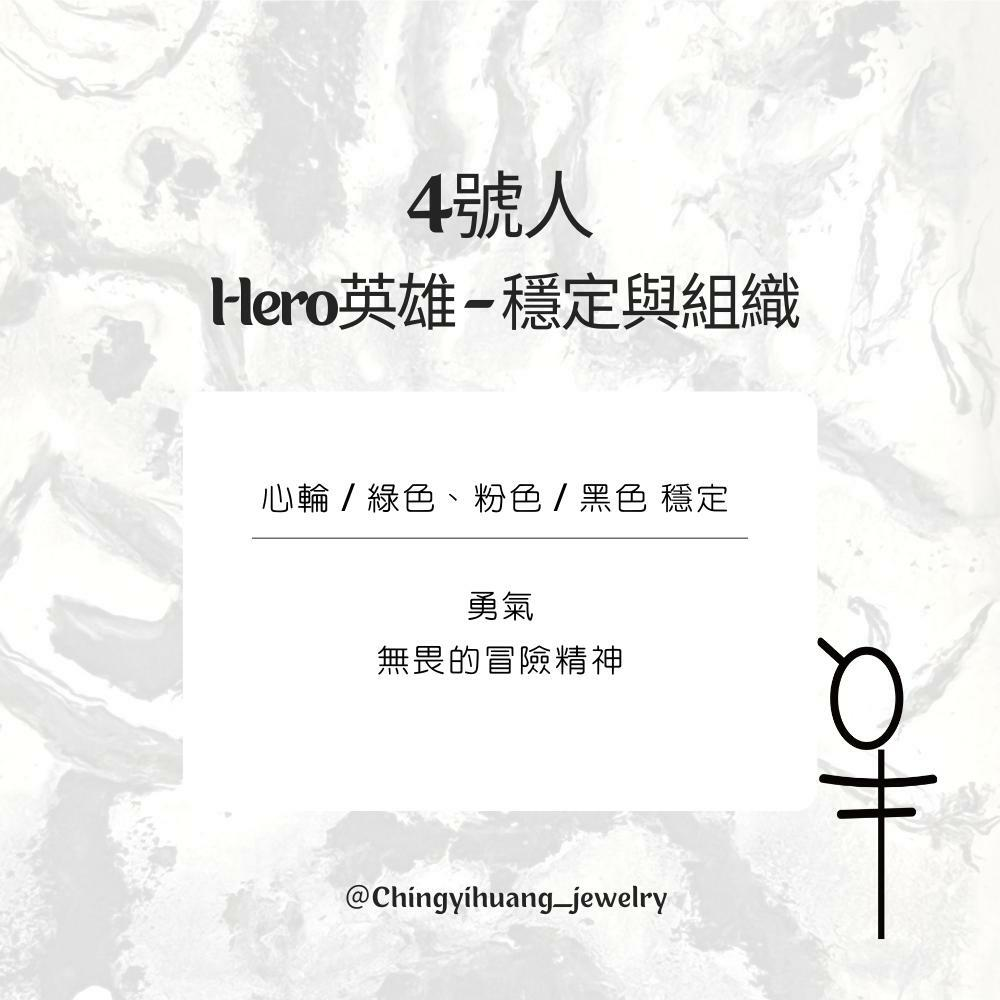4號人

Hero英雄 – 穩定與組織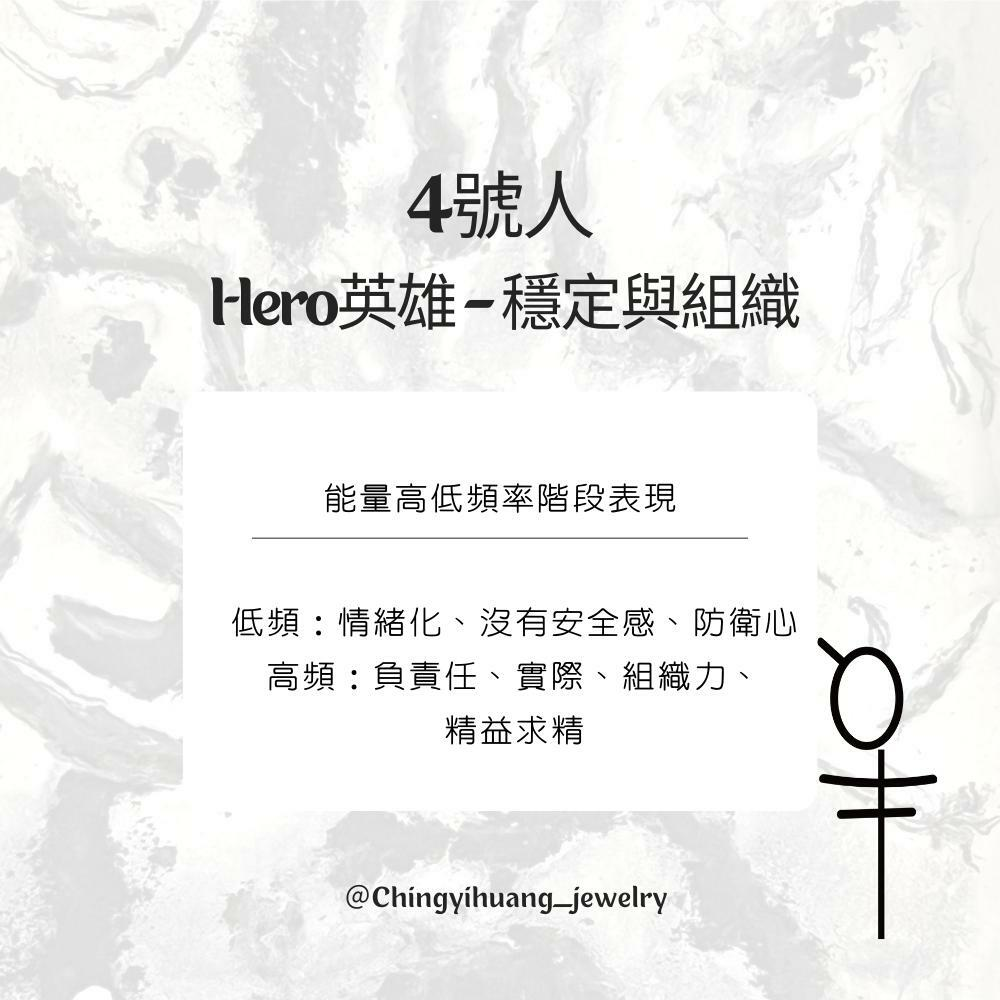🔳4號人 Hero英雄-穩定與組織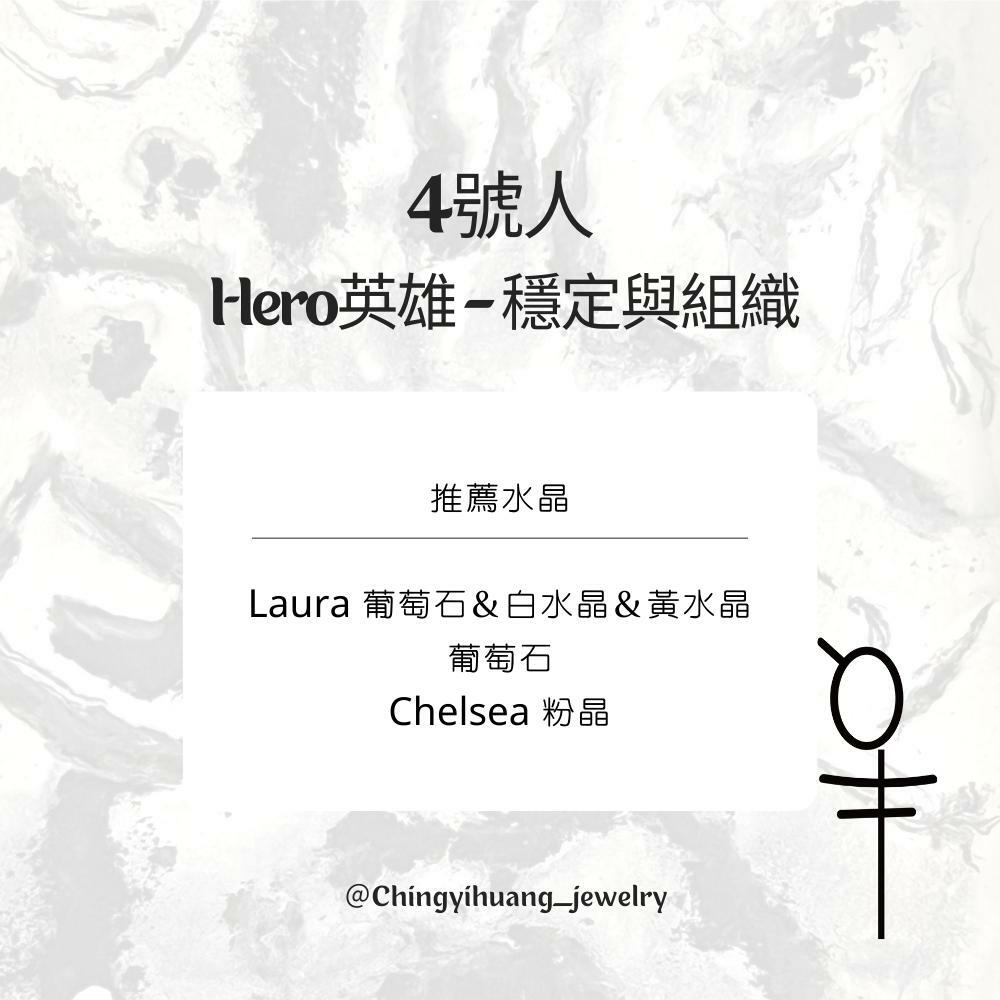CYH- Laura -葡萄石＆白水晶＆黃水晶 純銀 手環 (增加財富/淨化心靈/消除壓力/自信喜悅)

CYH- 巴西 玻璃體葡萄石 純銀 手環 (安定神經/助眠/消除壓力/緩解憤怒)

CYH-Chelsea-粉水晶 白水晶 純銀 手鍊/項鍊（ 招好桃花/ 情感升溫/ 增進人緣/ 加強心肺功能）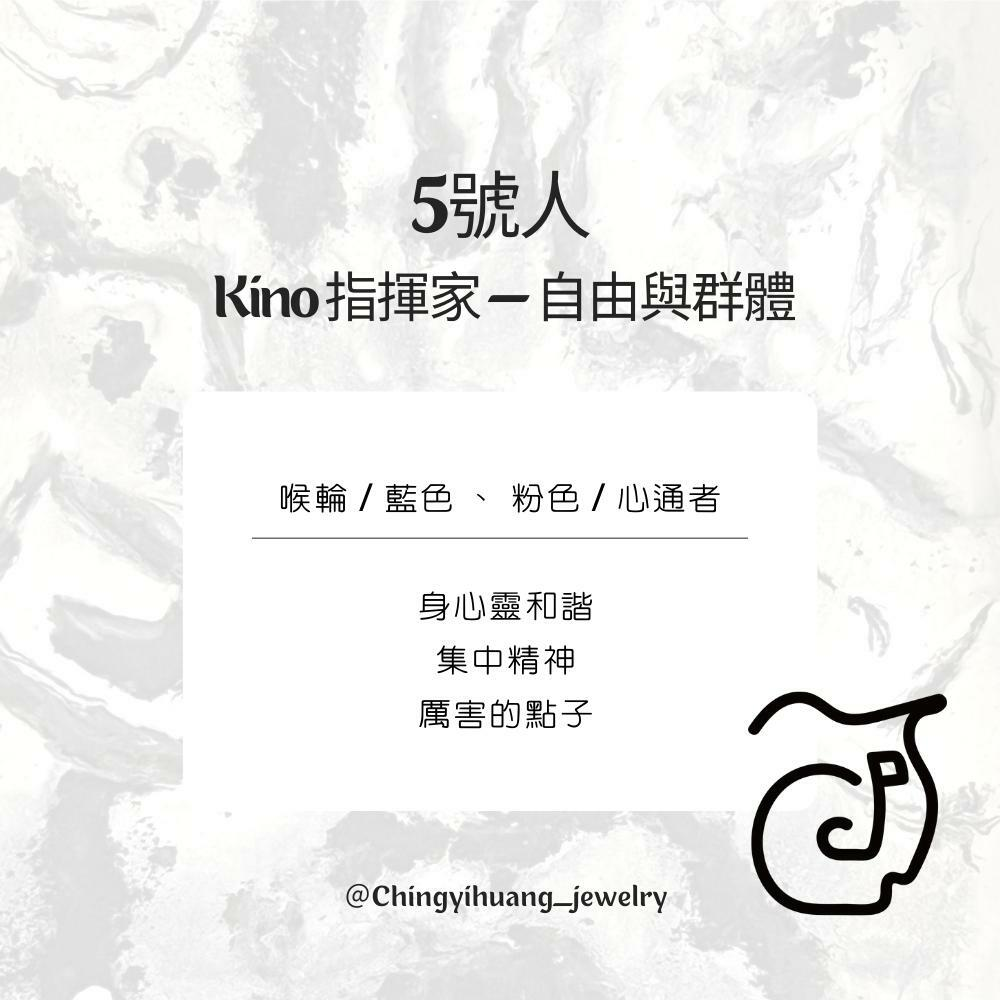5號人

Kino 指揮家 — 自由與群體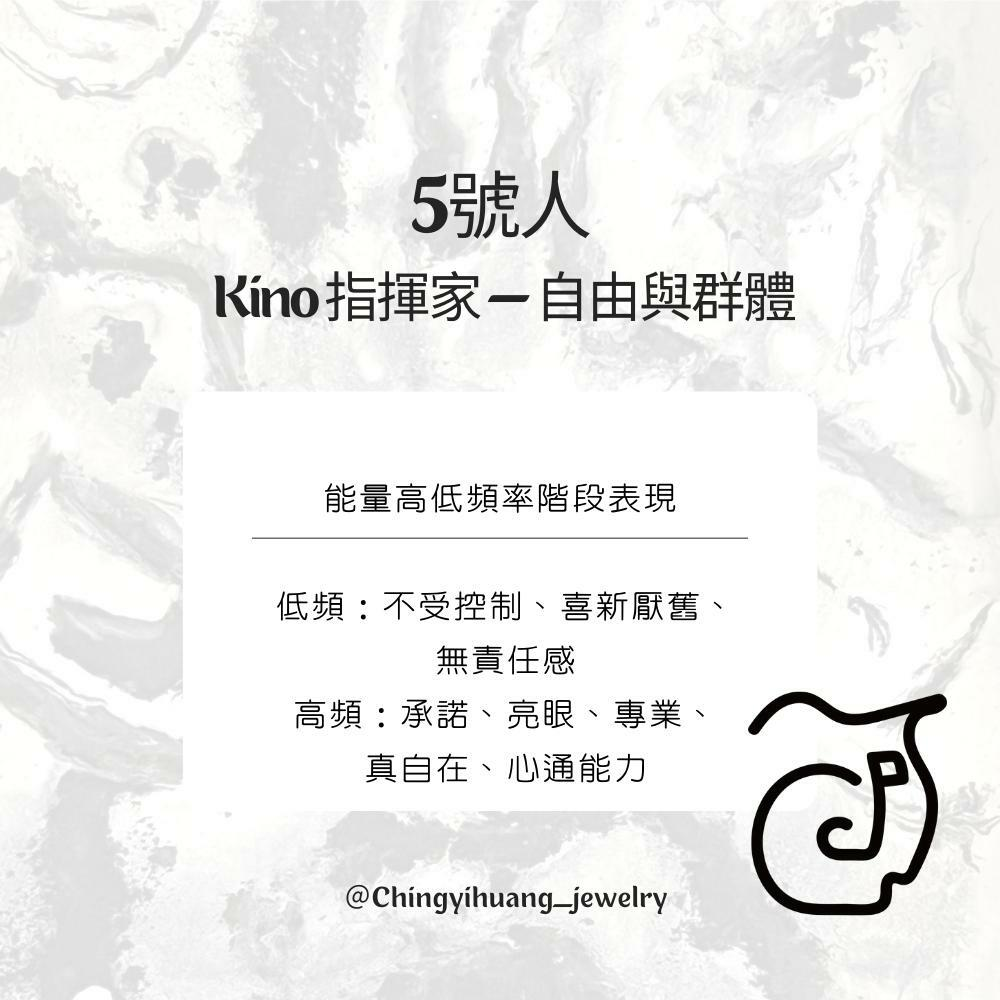🔳5號人 Kino 指揮家—自由與群體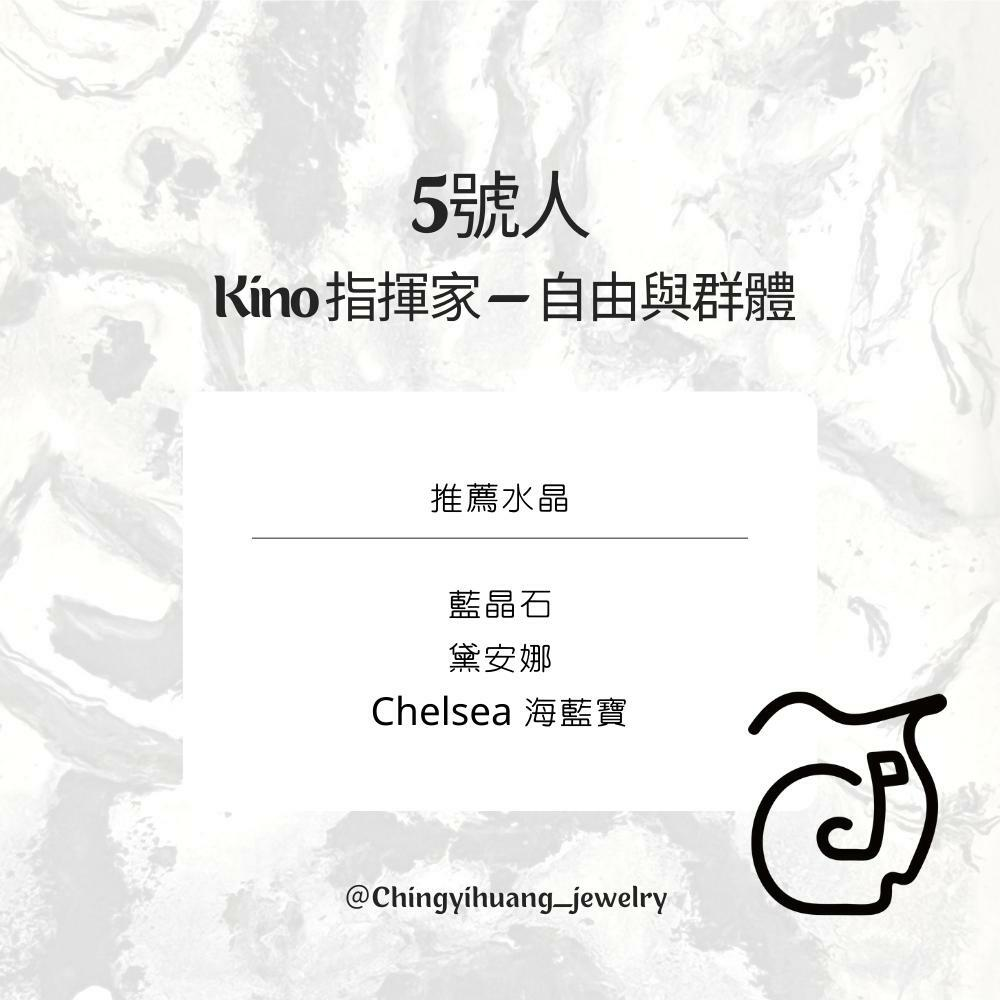CYH-天然 印度 方形 鑽切藍晶石 水晶手環（提升精神/洞察力/消除疲勞/活化溝通）

CYH- 天然 藍晶石 純銀扁珠 水晶手環（提升精神/洞察力/消除疲勞/活化溝通）

CYH- 釋放與療癒- 黛安娜 天然海藍寶/月光石/拉長石/白水晶 手環 （安神/ 助眠/ 平靜/ 釋放情緒/緩解焦慮）

CYH-Chelsea-海藍寶 白水晶 純銀 手鍊/項鍊（加強溝通表達/ 創造力 / 帶來積極正能量/ 冷靜沈著/提升自我價值）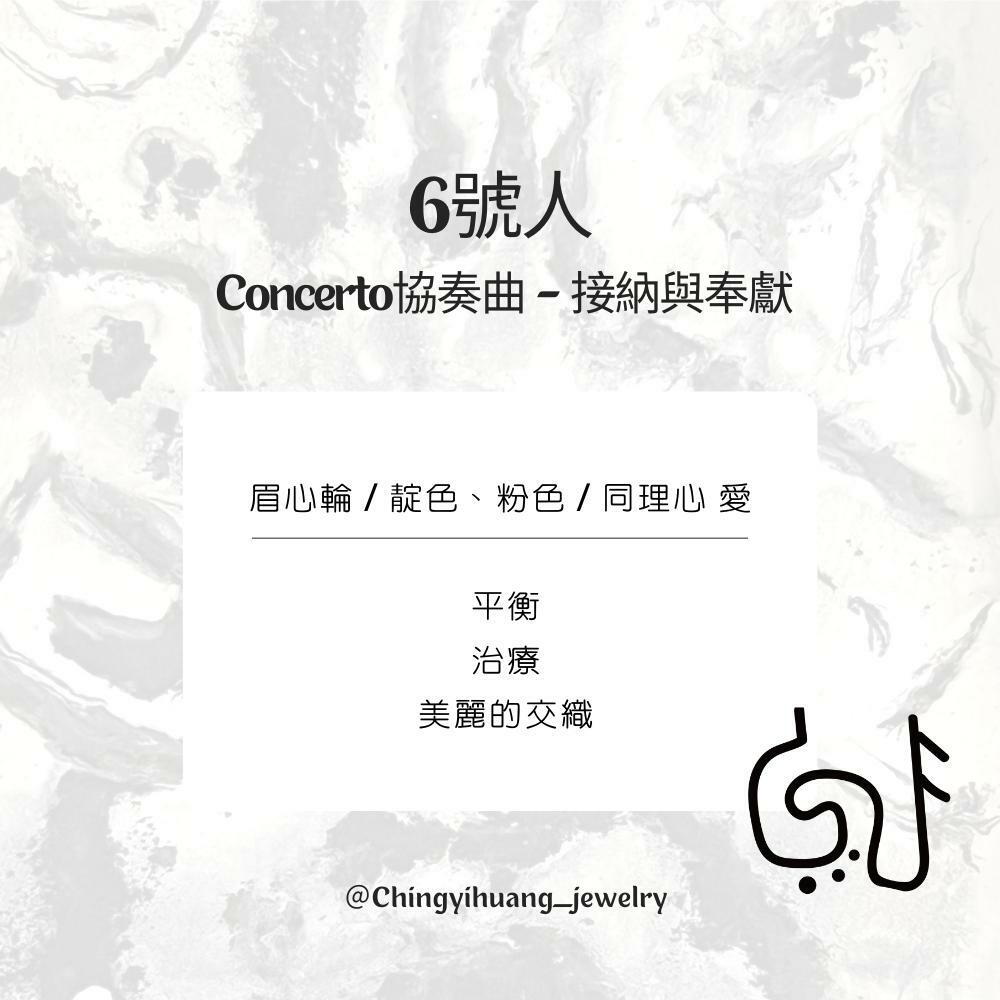6號人

Concerto協奏曲 – 接納與奉獻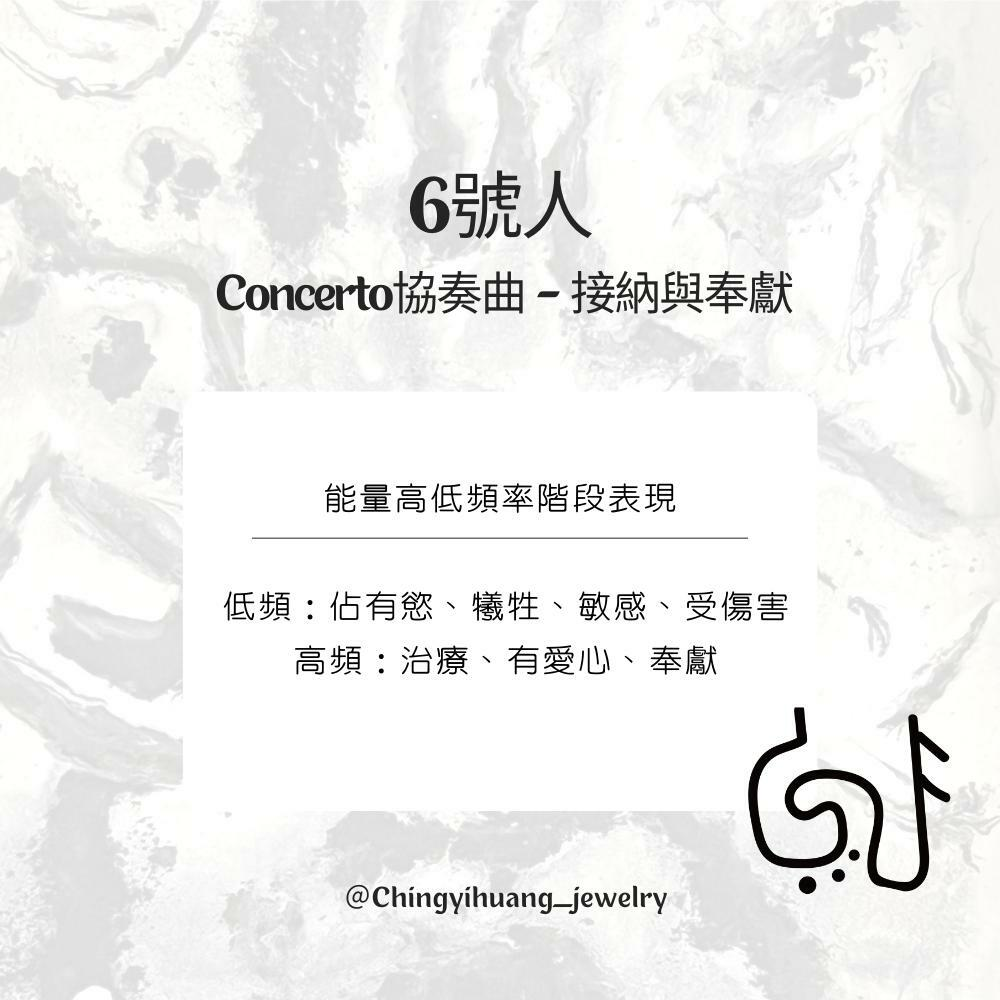🔳6號人 Concerto協奏曲-接納與奉獻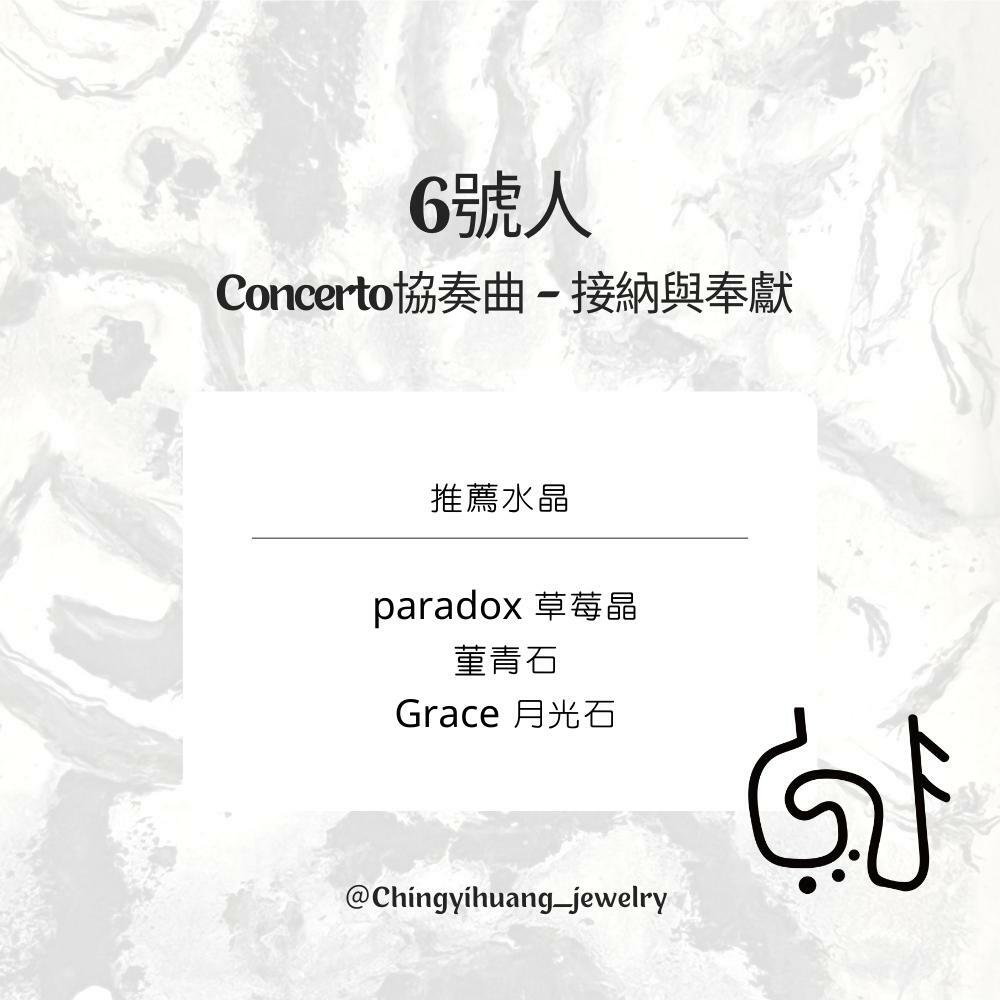CYH-Paradox-天然 不規則 草莓晶 純銀手鍊（ 招正桃花/增加魅力吸引力 /心情喜悅/ 行動積極）

CYH- 天然 菫青石 純銀 手環（突破現狀/轉念/取回自信/看透真相/找到方向）

CYH- 天然 菫青石 純銀扁珠 手環（突破現狀/轉念/取回自信/看透真相/找到方向）